# Two-Phase Flow Development of R134a in a Horizontal Pipe: Computational Investigation

Two-Phase Flow Development of R134a in a Horizontal Pipe: Computational Investigation

Raid A. MahmoodKhalid Saleh Veyan A. Musa Enass Massoud Ahmad Sharifian-Barforoush Lokman A. Abdulkareem

School of Mechanical and Electrical Engineering, University of Southern Queensland, Toowoomba 4350, Australia

Department of Mechanical Engineering, University of Zakho, Zakho City, P O Box 12, Kurdistan Region of Iraq, Iraq

Mechanical Engineering Department, Arab Academy for Science, Technology and Maritime Transport, Egypt

Department of Petroleum Engineering, University of Zakho, Zakho City, P O Box 12, Kurdistan Region of Iraq, Iraq

Institute of Fluid Dynamics, Helmholtz-Zentrum Dresden-Rossendorf, Dresden 01328, Germany

Corresponding Author Email:
Raid.Mahmood@usq.edu.au
Page:
1532-1540
|
DOI:
https://doi.org/10.18280/ijht.390515
9 June 2021
|
Accepted:
26 September 2021
|
Published:
31 October 2021
| Citation

OPEN ACCESS

Abstract:

To improve the performance of vapor compression refrigeration systems that use vertical gravitational flash tank separators, the liquid separation efficiency of the vertical gravitational flash tank separator requires to be approved. To approach this improvement, the two-phase flow development and its behavior after the expansion device need to be investigated and predicted. For thus, this paper presents a three-dimensional computational investigation of the two-phase flow development of R134a after the expansion device in a horizontal pipe. Computational Fluid Dynamic (CFD) was used to predict the two-phase development and its behavior in the horizontal pipe. ANSYS 16.2 program was used to generates the geometry of the three-dimensional horizontal pipe of 2 meters long and 25 mm inner diameter. The hexahedral mesh was generated and it is assessed to obtain the optimum mesh size and number. Eulerian-Eulerian two-phase model was used with k-ɛ turbulence model. R134a was used as a working fluid in the horizontal pipe utilizing four different inlet diameters: 12, 12.5, 25, and 50.0 mm. Mass flux and vapor quality have been changed from 288 to 447 kg/m2.s and from 10 to 20% respectively. Results were validated against experimental results from the literature and revealed that the separation region length is affected by the initial phase velocities, inlet vapor quality, and inlet tube diameter. An empirical correlation to predict the expansion region length is proposed as a function of Froude, Webber, and Lockhart-Martinelli numbers.

Keywords:

two-phase flow, R134a, computational fluid dynamic, expansion length, two-phase flow development, flow in a horizontal pipe

1. Introduction

Two-phase flow has been investigated in many studies due to the importance of such flow that represented in many applications [1-3]. Two-phase liquid-gas flow is involved in air conditioning and refrigeration systems [4, 5]. Two-phase flow pattern plays a significant role to distribute the refrigerant in headers where effective refrigerant distribution improves the heat transfer characteristic of the evaporators and condensers [6, 7]. The two-phase flow is generated after the expansion device, and investigated by many studies dealing with a two-phase flow characteristic in a horizontal tube; Awwad , Canière et al. , Bhramara, et al. , Dalkilic et al. , Ekambara et al. , Kondou et al. , Dasari et al. , Chen et al. , Becker et al. , Bottin at al. , Rana et al. , Duan et al.  Mahmood et al. . However, few studies were reported for the adiabatic R134a two-phase flow after the expansion device. Duan et al.  reported that in two-phase flows there are various flow patterns such as stratified, slug, annular, and dispersed flows, for different fluid properties and flow conditions. Therefore, two-phase flow patterns after the expansion valve, which is the inlet to the flash tank and/or evaporator, have a significant effect on the separation performance of the flash tank and refrigerant distribution in the evaporator . Mahmood et al.  considered the two-phase flow behavior in the gravitational flash tank separator using water-vapor two-phase flow. The results demonstrated that the liquid separation efficiency can be affected by the inlet two-phase flow behavior. Mahmood et al.  also reported that the inlet two-phase flow direction can influence the two-phase flow behavior and separation efficiency of a vertical separator. Tong  used a numerical approach to predict the two-phase flow behavior of water-oil flow in gravity separators. Saidj et al.  conducted an experimental study to investigate the behavior of air-water mixtures flowing through 90-degree bends. The results revealed that the void fraction increased with the gas superficial velocity and for the experimental condition, plug, slug, and stratified flow patterns occurred in the horizontal pipe while slug and churn flow patterns were presented in the vertical part. In the present study, CFD simulations were conducted to simulate the adiabatic R134a two-phase flow after the expansion valve using four internal diameters for horizontal tube (12.0, 12.5, 25.0, and 50.0 mm) and investigate the effects of the liquid droplets size, mass flux and vapor quality on the expansion length.

Bottin et al.  conducted an experimental study of adiabatic two-phase flow in a horizontal tube. Mixture of water and air have been used as working fluid. Flow pattern maps were recorded using high speed video. The results revealed that at certain distance which was defined as 20D from the inlet of the tube, the flow was separated into two zones: the lower region with very low gas void fraction and upper region with very high gas void fraction.

Zhao et al.  experiment the pressure effects on the hydrodynamic characteristic of gas-liquid two-phase flows in a T-junction micro channel. Nitrogen and de-ionized water are used as a working fluid to operate under a pressure range from 0.1 to 5 MPa. The gas weber number varies from 1.37 x 10-5 to 3.46 under atmospheric pressure and from 1.70 x 10-3 to 70.32 at elevated pressures. The results revealed that the operating pressure has significant effects on the two-phase flow behaviour. Most of the previous studies show the behaviour and flow pattern of the two-phase flow in a horizontal tube at the developed region. However, the expansion region length, which is located directly after the expansion valve in an adiabatic two-phase flow has not been considered yet. As it can be seen from the literature, many studies have considered the two-phase flow; however, using the R134a after expansion device still needs more investigation. Thus, the aim of this study is to simulate the adiabatic of R134a two-phase flow after the expansion valve using a horizontal tube with different diameters and investigate the liquid droplets size effects, mass flux and vapour quality on the expansion length.

2. Model Development

Figure 1 illustrates the geometry and flows passage of a horizontal pipe connected with an expansion valve considered for the present computational modeling. The geometry consists of a horizontal pipeline with an internal diameter and length of 50 mm and 2000 mm, respectively. Figure 1 presents the region of the single-phase before the expansion valve and the two-phase flow after the expansion valve. When the liquid of R134a is supplied through the inlet as a single-phase flow, the expansion process initiates a well-mixed two-phase flow with a slip ratio of 1.0 directly after the expansion device [27, 28]. This initial expansion region occupies a short distance directly after the expansion device . Following that, the fluid enters a non-stable developing stage where segregation starts under the effect of gravity . After a certain distance, the flow starts maintaining a stable volume fraction for both phases and enters the developed flow regime.

## 1.png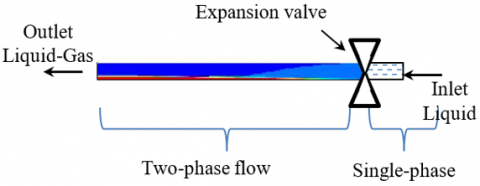Figure 1. Geometry and flow passage of a horizontal pipe

The computational control volume of the horizontal tube under investigation was generated by design modular of ANSYS as presented in Figure 2. The geometry was taken built using an inside diameter is 25 mm and the length of the circular tube is 2000 mm. To reduce the computational time, the domain represents half of the physical experiment with the symmetry along the vertical plane being exploited.

## 2.png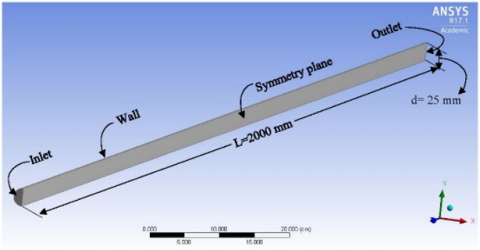Figure 2. Computational control volume for the horizontal pipe

3. Simulation Approach

Eulerian-Eulerian two-phase model is the most complex of the two-phase models; however, it is more accurate than other models because it solves a set of n two-phase differential equations for each phase , where the computational effort depends strongly on the number of transport equations which need to be solved. Eulerian-Eulerian two-phase models have been used to simulate two-phase flows in different geometries: Hwang and Pal , Roul and Sahoo , Delnoij et al. , Rampure et al.  Gandhi et al. , Dasari and Goshika , Walvekar et al. , Parvareh et al.  and Ekambara et al. .

To present two-phase flow equations, q and p will be used to describe liquid and gas phases respectively. The general form of the two-phase governing model can be presented as follows.

$\frac{\partial}{\partial t}\left(\alpha_{q} \rho_{q}\right)+\nabla .\left(\alpha_{q} \rho_{q} v_{q}\right)=\sum_{p=1}^{n}\left(m_{p q}-m_{q p}\right)+S_{q}$,    (1)

Bowers , defined the volume fraction for the individual phase as the volume occupied by that phase. So, the volume of the phase q can be given by:

$V_{q}=\int \alpha_{q} d V$,    (2)

$\sum_{q=1}^{n} \alpha_{q}=1$.    (3)

Accordingly, the volume fraction of the gas phase can be given by:

$\alpha_{q}=\frac{Q_{q}}{Q_{t}}=\frac{V_{q}}{V_{t}}$,    (4)

According to the FLUENT program , the Conservation of momentum can be written as:

$\frac{\partial}{\partial t}\left(\alpha_{q} \rho_{q} v_{q}\right)+\nabla .\left(\alpha_{q} \rho_{q} v_{q} v_{q}\right)=-\alpha_{q} \nabla p+\nabla \cdot \tau_{q}+$

$\alpha_{q} \rho_{q} g+\sum_{p=1}^{n}\left(R_{p q}+m_{p q} v_{p q}-m_{q p} v_{q p}\right)+$

$\left(F_{q}+F_{l i f t, q}+F_{v m, q}\right)$.    (5)

where, $\tau_{q}$ is the q phase stress-strain tensor, given by:

$\tau_{q}=\alpha_{q} \mu_{q}\left(\nabla . v_{q}+\nabla . v^{T}{ }_{q}\right)+\alpha_{q}\left(\lambda_{q}-\frac{2}{3} \mu_{q}\right) \nabla . v_{q} I .$    (6)

In Eq. (6) above, $\lambda_{q}$ and $\mu_{q}$ are the shear and bulk viscosity of phase q, $R_{p q}$ is an interaction force between phases, is the pressure shared by all phases and $p$ is the interphase velocity which can be defined as: $v_{p q}=v_{p}$; for $m_{p q}$> 0 and $v_{p q}$=$v_{q}$ for $m_{p q}$< 0, likewise, if  $m_{q p}$> 0 then $v_{q p}$=$v_{q}$, and if $m_{q p}$<0 then $v_{q p}$ = 0.4 [37, 38].

The momentum equation has to be closed with appropriate expression for the inter-phase force $R_{p q}$. This force depends on the pressure, friction, and cohesion in addition to other effects. This closure can be satisfied by: $R_{p q}$= $-R_{q p}$ and $R_{q q}$=0. To achieve a correct solution, a simple iteration is available which can be defined as:

$\sum_{p=1}^{n} R_{p q}=\sum_{p=1}^{n} K_{p q}\left(v_{p}-v_{q}\right)$,    (7)

The lift force, shown in Eq. (5), acts on the second phase particles, droplets or bubbles, under the velocity gradients. The lift force becomes significant when the particle size is large, however, the assumption, in the numerical model solution, that the particle size is much smaller than the inter-particle spacing may lead to the exclusion of the lift forces from Eq. (5). Terzuoli et al.  defined the lift force acting on a secondary phase p in a primary phase q as:

$F_{\text {lift }}=-0.5 \rho_{q} \alpha_{q}\left(v_{q}-v_{p}\right)\left(\nabla . v_{q}\right)$.    (8)

Virtual mass force is another force that is included in the momentum equation. It acts on the two-phase flow when the secondary phase p accelerates relative to primary phase q . Accordingly, the effect of virtual mass force will be significant when the density of the second phase is much smaller than the density of the primary phase. The virtual mass force can be presented as follows:

$F_{v m}=0.5 \alpha_{q} \rho_{q}\left(\frac{d_{q} v_{q}}{d t}-\frac{d_{q} v_{p}}{d t}\right)$.    (9)

The derivative in Eq. (9) is the phase material derivative which can be presented as :

$\frac{d_{q}(\emptyset)}{d t}=\frac{\partial(\emptyset)}{\partial t}+\left(v_{q} . \nabla\right) \emptyset$,    (10)

$\frac{d_{p}(\emptyset)}{d t}=\frac{\partial(\emptyset)}{\partial t}+\left(v_{p} . \nabla\right) \emptyset$.    (11)

The modelling of turbulence in multiphase flow is extremely complex compared to a single-phase [38, 40]. Kerdouss et al.  reported that the k-ɛ model is appropriate for three-dimensional two-phase flow prediction because it employs transport equations that can be solved for kq and ɛq which are kinetic energy and the dissipation rate for phase q respectively. Furthermore, the k-ɛ per each phase is also appropriate when the turbulence transfer among the phases plays a dominant role. Therefore, in the present study, k-ɛ turbulence model has been selected to simulate the two-phase turbulent behaviour for each phase. The k-ɛ is represented in Equations 12 and 13 ;

$\frac{\partial}{\partial t}\left(\alpha_{q} \rho_{q} k_{q}\right)+\nabla \cdot\left(\alpha_{q} \rho_{q} U_{q} k_{q}\right)=\nabla \cdot\left(\alpha_{q}\left(\mu_{q}+\right.\right.$

$\left.\left.\frac{\mu_{t, q}}{\sigma_{k}}\right) \nabla k_{q}\right)+\left(\alpha_{q} G_{k, q}-\alpha_{q} \rho_{q} \varepsilon_{q}\right)+\sum_{p=1}^{N} K_{p q}\left(C_{p q} k_{p}-\right.$

$\left.C_{q p} k_{q}\right)-\sum_{p=1}^{N} K_{p q}\left(U_{p}-U_{q}\right) \cdot \frac{\mu_{t, p}}{\alpha_{q} \sigma_{q}} \nabla \alpha_{p}+$

$\sum_{p=1}^{N} K_{p q}\left(U_{p}-U_{q}\right) \cdot \frac{\mu_{t, p}}{\alpha_{q} \sigma_{q}} \nabla \alpha_{q}$,    (12)

$\frac{\partial}{\partial t}\left(\alpha_{q} \rho_{q} \varepsilon_{q}\right)+\nabla \cdot\left(\alpha_{q} \rho_{q} U_{q} k \varepsilon_{q}\right)=\nabla \cdot\left(\alpha_{q}\left(\mu_{q}+\right.\right.$

$\left.\left.\frac{\mu_{t, q}}{\sigma_{k}}\right) \nabla \varepsilon_{q}\right)+\frac{\varepsilon_{q}}{k_{q}}\left[C_{1, \varepsilon} \alpha_{q} G_{k, q}-C_{2, \varepsilon} \alpha_{q} \rho_{q} \varepsilon_{q}+\right.$

$C_{3, \varepsilon}\left(\sum_{p=1}^{N} K_{p q}\left(C_{p, q} k_{p}-C_{q, p} k_{q}\right)\right)-\sum_{p=1}^{N} K_{p q}\left(U_{p}-\right.$

$\left.\left.U_{q}\right) \cdot \frac{\mu_{t, p}}{\alpha_{q} \sigma_{q}} \nabla \alpha_{p}+\sum_{p=1}^{N} K_{p q}\left(U_{p}-U_{q}\right) \cdot \frac{\mu_{t, q}}{\alpha_{q} \sigma_{q}} \nabla \alpha_{q}\right]$.    (13)

where, $C_{p, q}$ and $C_{q, p}$ can be obtained from:

$C_{p, q}=2$,    (14)

$C_{q, p}=2\left[\frac{\tau_{p, q}}{1+\tau_{p, q}}\right]$.    (15)

4. Boundary Conditions

To simulate and solve the numerical equations, boundary conditions need to be set. The symmetry boundary condition is selected to reduce the computational effort to half; therefore, reducing the computational time. The Symmetric plane is chosen at the vertical diameter of the pipe. At the inlet of the pipe, the velocity inlet is used to define the velocity of each phase. A uniform velocity across the pipe cross-section is used in all numerical simulations because the region after the expansion valve is assumed a homogeneous region and there is no slip velocity ratio between both phases. The stationary no-slip boundary condition is imposed at the walls of the pipe which can be presented as the equation below,

$u_{\text {wall }}=k_{\text {wall }}=0$.    (16)

At the outlet of the pipe pressure outlet is selected as the boundary condition. Figure 3 shows the schematic of boundary conditions.

## 3.pngFigure 3. Schematic of boundary conditions

5. Simulation Procedure

In the present numerical simulation study, the FLUENT 17.1 program was used. FLUENT provides different models to predict the two-phase flow behaviors. A Eulerian-Eulerian approach is one of the two-phase models. The Eulerian-Eulerian approach is more reasonable than other numerical approaches to predict the two-phase flow behavior after the expansion valve because the flow is dense . The Eulerian-Eulerian approach also deals with the second phase directly after expansion as a continuous phase because the flow is homogenous and mixed very well . Therefore, the Eulerian-Eulerian model, pressure-based solver, and steady time have been used to simulate three-dimensional adiabatic two-phase flows. R134a has been used as a working fluid and four diameters: 12mm, 12.5mm, 25mm, and 50mm have been used in the numerical simulation. To present the CFD simulation results for the two-phase flow in a horizontal tube, three test lines were defined to capture the liquid volume fraction distribution at 0.5, 1, and 1.5 mm from the top of the tube at the upper section as shown in Figure 4. According to the volume fraction definition, the two-phase flow becomes a single-phase pure liquid when the liquid volume fraction is equal to one. While, when the liquid volume fraction is equal to zero, the two-phase flow becomes a pure single vapor phase. Therefore, the volume fraction distribution along the pipe is used to define the two-phase flow behavior and liquid separation.

## 4.png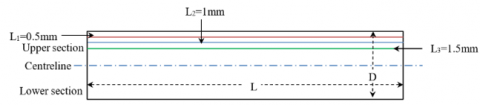Figure 4. Drawing to show three test lines: 0.5, 1and 1.5mm from the top of the pipe which are colored by red, blue and green respectively

6. Grid Independent Study

In the present work, a grid-independent study has been investigated. Five computational grids of 30600, 47740, 80558, 142000, and 224400 elements of mesh were used to identify the optimum grid size for two-phase computational simulation. To achieve the study of grid independence, boundary conditions, (G288 kg/m2.s and x 10%), of one case study was used in the grid-independent study simulation. The boundary condition includes velocity inlet at the inlet of a horizontal pipe and pressure outlet at the outlet of the pipe. ANSYS 16.2 was used to create the hexahedral mesh which has benefits such as reducing the computational time and improvement in the quality of the mesh by comparison with tetrahedron mesh .

Figure 5(a) shows liquid volume fraction distribution along the pipe at 1.5 mm from the top for the five different numbers of meshes. It can be seen that there is no significant change in the liquid volume fraction when the mesh number increased beyond 142000. Figure 5(b) shows the minor difference between 142000 and 224400 which is found to be within 0.4% average difference. In addition, the liquid volume fraction contours for 224400, 142000, and 30600 computational grids are presented in Figure 6. In this figure, the red color represents the liquid phase, while the blue color represents the vapor phase. In Figure 6, the stratified flow with 142000 cells is depicted very well, and the interface between the liquid and vapor phases is captured in the developed region (1000 mm from inlet), compared with a lower number of cells at the same position. In addition, the range of the parameters for mesh quality were considered to select the optimum mesh number. The orthogonal quality ranges from 0 to 1, 0 represents low mesh quality. The minimum orthogonal quality should always be greater than or equal to 0.01 Mahmood . The aspect ratio is relevant to the wall function and should be small enough to allow the solution to capture the flow details near the wall. The skewness value, which is inversely related to solution accuracy, should be small enough to minimize error in the solution. For the meshes used in the present simulations, the orthogonal quality was 0.9 with a minimum value of 0.07, the aspect ratio was 3.56, and skewness value was 0.26. These values indicate that according to the established criteria, good mesh qualities were obtained and used in the present simulations. Therefore, based on the liquid volume fraction contour results and average difference with 224400, 142000 cells are selected as the appropriate number of cells to be used in the simulations.

## 5a.png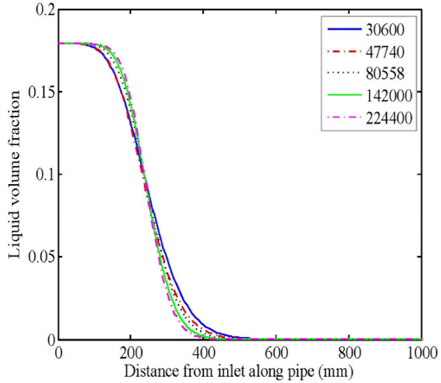(a) Liquid volume fraction at top of the pipe (1.5 mm from top) at 288 kg/m2.s and 10% vapor quality

## 5b.png(b) Liquid volume fraction at top of the pipe (1.5 mm from top) at 288 kg/m2.s and 10% vapor quality

Figure 5. Liquid volume fraction at top of the pipe

## 6.png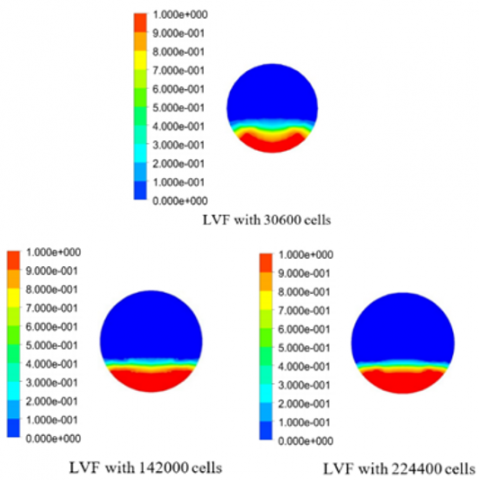Figure 6. Liquid volume fraction at 288 kg/m2.s mass flux and 10% at developed region (1000 mm from inlet)

7. Validation of CFD Simulation

Experimental data from Fei  was selected to validate the numerical results. Figure 8 shows the experimental result at 12mm inlet diameter, 20% vapor quality, 265 kg/m2.s mass flux, and 250-micron droplets diameter. In FLUENT 16.2, the same geometry and boundary conditions had been used by Fei and Hrnjak  were used just for validation purposes. In addition, to better check and compare the results, three test lines: 0.5, 1, and 1.5 mm from the top of the pipe have been used for capturing the liquid volume fraction on the top. In Figure 7, the expansion region length was experimentally identified by Fei and Hrnjak , according to a significant change in the number of droplets at the top. This occurred at 107.5mm from the inlet. In CFD simulation results, the liquid volume fraction along the top of the pipe is assessed to identify the significant separation. To ensure consistency, a 0.005 liquid volume fraction is considered at three top lines: 0.5, 1, and 1.5mm from the top as shown in Figure 8.

## 7.png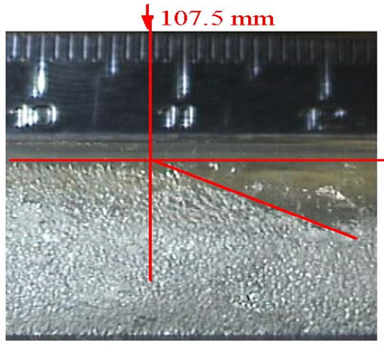Figure 7. Experimental data at 12 mm inlet tube diameter, 265 kg/m2.s, and 250 microns had been done by Fei and Hrnjak 

## 8.png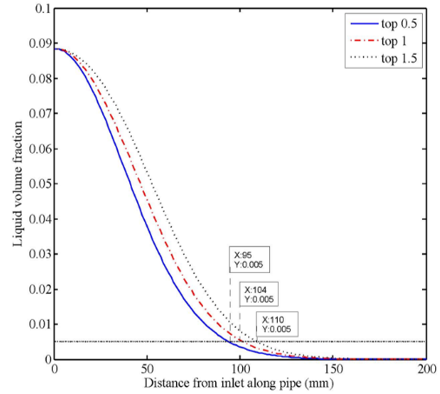Figure 8. CFD simulation prediction for 12mm inlet tube diameter, 265 kg/m2.s and 250 microns

From the comparison of the CFD results with experimental results in Figure 7, and Figure 8, the liquid volume fraction along lines 1 and 1.5 from the top has an acceptable agreement with the experimental result.

8. Results and Discussion

8.1 Effect of the mass flux on the liquid volume fraction

To focus on the effect of mass flux on the two-phase flow development and phase separation, the droplet size is fixed at 250-micron and vapor quality at 20% in a 50 mm inlet tube diameter and 2 m in length. Figure 9 shows the effect of mass flux on the phase separation at the 1.5 mm test line from the top of the pipe. The simulation results show that the expansion length increased when the initial velocities increased because of increased the momentum of liquid droplet. The CFD predicts expansion region length for the 288kg/m2.s, 335kg/m2.s and 447kg/m2.s; 348mm, 405mm, and 543mm respectively.

## 9.png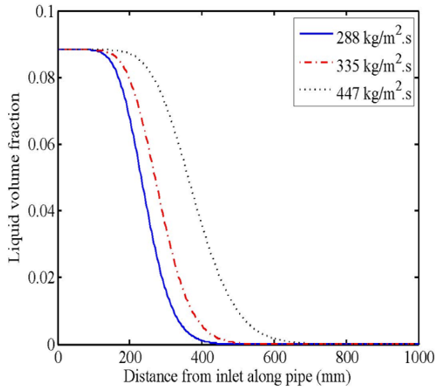Figure 9. Liquid volume fraction along the tube at 50mm inlet diameter, 20% vapor quality, and 250 µm droplets diameter at the top (1.5mm from top)

8.2 Quality effect on the liquid volume fraction

In order to present the vapor quality effect on the liquid volume fraction, all the other parameters were fixed, (288 kg/m2.s mass flux, 2 m long, 12.5 mm inlet diameter, and 250 micron droplet size). Figure 10 shows the relationship between vapor quality and flow development at 1.5 mm from the top along the tube length. The figure shows that the length of the expansion region is affected by the vapor quality. When the inlet vapor quality is increased, the liquid separation is delayed. The CFD predicts the expansion length of the expansion region for the three values of quality 10%, 15%, and 20%; 60.2mm, 78.39mm, and 95.19mm respectively.

## 10.png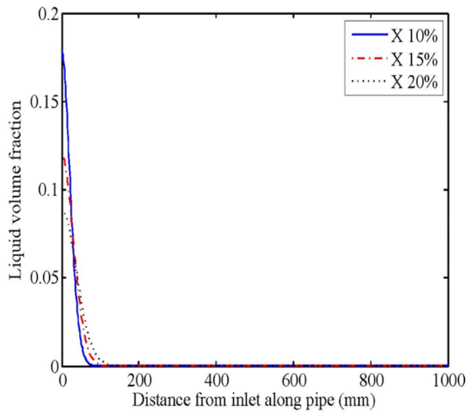Figure 10. Liquid volume fraction along the tube at 12.5mm inlet diameter, 288 kg/m2.s mass flux, and 250 µm droplets diameter at the top of the pipe (1.5mm from top)

8.3 Inlet diameter effect on the liquid fraction

Four inlet tube diameters are tested: 12, 12.5, 25- and 50.0-mm. Figure 11 shows the inlet diameter effect on the flow development along the tube after expansion valve at 1.5 mm from the top of the tube. To study the diameter’s change effect, all other parameters were fixed at mass flux 288 kg/m2.s, 20% vapor quality, 250-micron droplet size, and 2 m pipe length. The CFD results show that there is little influence of the inlet diameter on the transition length between the expansion and developing regions for the small pipe diameters. However, the influence is significant when the diameter is 50.0 mm. Indeed, the expansion region lengths for 12mm, 12.5mm, 25.0mm, and 50.0mm were predicted by CFD of 95.19mm, 98.79mm, 120mm and 348mm respectively from the inlet of the pipe.

## 11.png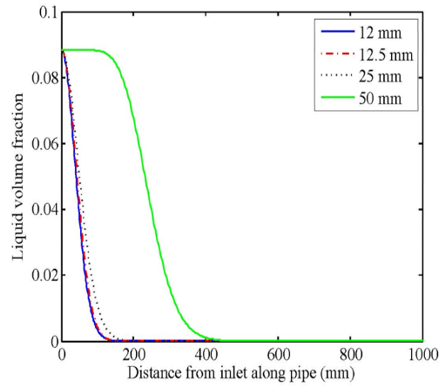Figure 11. Diameter effect on the flow development and liquid separation at 20% vapor quality, 250 µm droplets diameter, and 288 kg/m2.s mass flux at the top (1.5mm from top)

8.4 Droplet diameter effect on the liquid volume fraction

Figure 12 shows the CFD results for the phase separation when the liquid droplet diameter change. 50.0 mm inlet diameter and 2 m long at constant mass flux, 447 kg/m2.s, and 20% vapor quality were used as operating conditions to investigate the liquid dimeter effect. The numerical results revealed that smaller droplets diameter give longer expansion lengths. The CFD predicted the expansion region length for the; 250, 350, and 450 microns droplets; 543, 495, and 472 mm respectively from the inlet. This is consistent with the physics as the lower inertia of the droplets allows the flow to carry them for a longer distance before separation.

## 12.png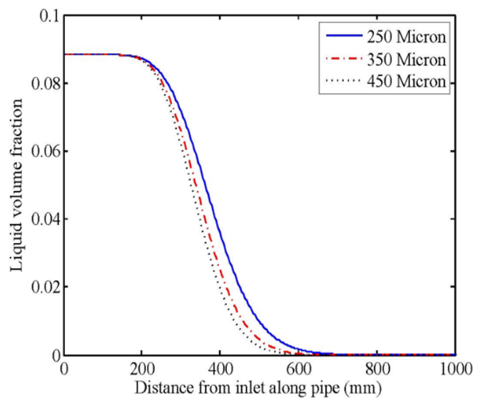Figure 12. Liquid droplet diameter effect on the flow development and liquid separation in 50 mm inlet diameter, 20% vapor quality, and 447 kg/m2.s mass flux at 1.5mm from the top

8.5 Empirical correlation for the expansion region length

In a horizontal tube after an adiabatic sudden expansion, homogenous, developing, and developed regions are generated. In the developed region, the flow maintains its characteristics along the tube . Liquid droplets start to move to the bottom of the pipe in a gas-liquid two-phase flow due to gravity and reduction of liquid velocity, So, to present the expansion region length, an empirical correlation to characterize the separation length, where the liquid is completely separated, three dimensionless numbers are used to present a proposed correlation for the current study, as shown in Eq. (17).

$x=27.9052 X_{t t}{ }^{0.2059} W e^{0.9558} \mathrm{Fr}^{-0.0254}$.    (17)

where, Xtt is Lockhart-Martinelli, this number presents vapor and liquid phases force interaction in two-phase flow and can be used to identify the two-phase flow pattern [41-43]. It can be defined as follows :

$\mathrm{X}_{\mathrm{tt}}=\left(\frac{\rho_{\mathrm{g}}}{\rho_{\mathrm{l}}}\right)^{0.5}\left(\frac{\mu_{\mathrm{l}}}{\mu_{\mathrm{g}}}\right)^{0.1}\left(\frac{1-\mathrm{x}_{\mathrm{in}}}{\mathrm{x}_{\mathrm{in}}}\right)^{0.9}$.    (18)

Webber number (We) is the ratio between the inertia force and surface tension force and defined as follows .

$\mathrm{We}=\frac{\rho_{\mathrm{g}} \mathrm{u}_{\mathrm{g}}^{2} \mathrm{D}}{\sigma}$.    (19)

Froude number (Fr) presents the ratio of inertia to gravity forces and defined as follows;

$\mathrm{Fr}=\frac{\mathrm{u}_{\mathrm{g}}^{2}}{\mathrm{gD}}$.    (20)

Figure 13 summaries the effect of the mass flux, tube diameter, vapor quality, and droplets diameter on the expansion region lengths. As can be seen, there is a positive effect between the expansion region length and mass flux, vapor quality, and tube diameter, but it is negative with the droplet diameter. Therefore, the expansion region will not be long when the droplet dimenter increased.

## 13.png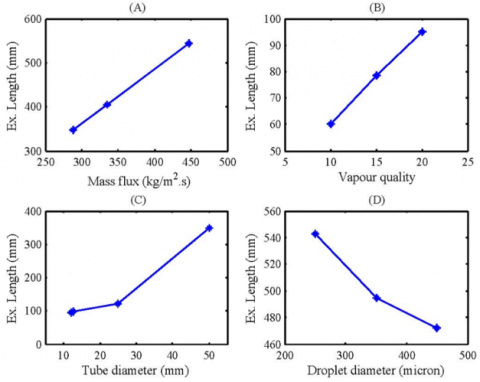Figure 13. Expansion length behaviour with: (A) mass flux variation at x20%, D 5cm and dm 250 micron, (B) vapour quality variation at D1.25cm, G 288 kg/m2.s and dm 250 micron, (C) tube diameter variation at G 288kg/m2.s x20%, dm 250 micron and (D) droplet diameter variation at G447 kg/m2.s D 5cm and x20%

9. Conclusions

An adiabatic two-phase liquid-gas flow has been investigated numerically using computational fluid dynamic (CFD). FLUENT 14.5 code has been used for predicting the flow behavior after the expansion valve in a horizontal pipe. The numerical results in terms of the two-phase separation are presented and discussed. Prediction of the expansion region length after the expansion valve and two-phase flow behavior pattern was in good agreement with experimental data from the literature.

Increasing the mass flux leads to a longer expansion region due to higher initial velocity, as shown in Figures 9 and 10, the expansion region length for 288 kg/m2.s,335 kg/m2.s and 447 kg/m2.s are 348 mm, 405 mm and 543 mm respectively. This results from the gravity liquid separation from the two-phase flow happening later.

Vapor quality at the inlet has a significant effect on the expansion region. The length of the expansion region is longer for higher quality, as there is a delay in liquid separation. The expansion region length for 10%, 15%, and 20% are 60.2 mm, 78.39 mm, and 95.19 mm respectively.

Inlet diameters: 12 mm, 12.5 mm, and 25 mm have little influence on the expansion region length. However, the influence is significant on phase separation for the 50 mm diameter. The expansion region lengths for the four diameters are 95.19 mm, 98.79 mm, 120 mm, and 348 mm respectively.

At large droplet diameter, there is no significant effect on the expansion region length, but there is a significant delay in liquid separation when the droplet size is less than 250 microns.

An empirical correlation for the stratified flow as a function of Fr and Xtt was proposed $\left(E x=27.9052 X_{t t}{ }^{0.2059} W e^{0.9558} \mathrm{Fr}^{-0.0254}\right)$ based on the numerical results for the refrigerant in the horizontal tube. It can be used to identify the expansion region length which would be useful to improve the two-phase flow distribution in headers and/or evaporators. It will also be a start point for future work to improve the two-phase flow distribution and development.

Nomenclature
 D tube diameter, mm dm liquid droplet diameter, micron Ex expansion region length, mm Fq the external body force, N Flift,q lift force, N Fvm,q virtual mass force, N G mass flux, kg.m-1. s-1 g gravity, m/s2 Kpq inter-phase momentum coefficient mpq mass transfer from phase q to phase p, kg/s mqp mass transfer from phase p to phase q, kg/s Qt total volume flow rate, m3/s Qq volume flow rate for phase, m3/s Rpq the interaction force between phases, N Sq source term which can be specified as constant Uq,Ul phase weighted velocities, m/s vq the velocity of phase q, m/s x vapor quality Greek symbols a void fraction σq the surface tension of phase. N.m-1 ρl liquid density, kg.m-3 $\rho_{g}$ gas density, kg.m-3 $\rho_{t}$ Liquid density, kg.m-3 $\mu_{g}$ vapour dynamic viscosity, kg. m-1.s-1 $\mu_{l}$ Liquid dynamic viscosity, kg. m-1.s-1 $\tau_{q}$ stress-strain tensor of q phase, N.m-2 $\lambda_{q}$ Shear of phase, N.m-2
References

 Musa, V.A., Mahmood, R.A., Khalifa, S.M.N., Ali, O.M., Abdulkareem, L.A. (2021). Flow patterns of oil-gas and pressure gradients in near-horizontal flow pipeline: Experimental investigation using differential pressure transducers. International Journal of Heat and Technology, 39(2): 621-628. https://doi.org/10.18280/ijht.390233

 Abdulkareem, L.A., Musa, V.A., Mahmood, R.A., Hasso, E.A. (2021). Experimental investigation of two-phase flow patterns in a vertical to horizontal bend pipe using wire-mesh sensor. Revista de Chimie, 71(12): 18-33. https://doi.org/10.37358/rc.20.12.8383

 Musa, V.A., Abdulkareem, L.A., Ali, O.M. (2019). Experimental study of the two-phase flow patterns of air-water mixture at vertical bend inlet and outlet. Engineering, Technology & Applied Science Research, 9(5): 4649-4653. https://doi.org/10.48084/etasr.3022

 Srisomba, R., Mahian, O., Dalkilic, A.S., Wongwises, S. (2014). Measurement of the void fraction of R-134a flowing through a horizontal tube. International Communications in Heat and Mass Transfer, 56: 8-14. https://doi.org/10.1016/j.icheatmasstransfer.2014.04.004

 Mahmood, R.A. (2020). Case study of liquid suction heat exchanger in a mechanical refrigeration system using alternative refrigerants. International Journal of Engineering & Technology, 9(3): 644-649. https://doi.org/10.14419/ijet.v9i3.30777

 Chen, H., Xu, J., Li, Z., Xing, F., Xie, J. (2012). Stratified two-phase flow pattern modulation in a horizontal tube by the mesh pore cylinder surface. Applied Energy, 112: 1283-1290. https://doi.org/10.1016/j.apenergy.2012.11.062

 Hrnjak, P. (2004). Developing adiabatic two phase flow in headers - distribution issue in parallel flow microchannel heat exchangers. Heat Transfer Engineering, 25(3): 61-68. https://doi.org/10.1080/01457630490280128

 Mahmood, R.A., Buttsworth, D., Malpress, R. (2019). Computational and experimental investigation of using an extractor in a vertical gravitational flash tank separator. International Journal of Automotive and Mechanical Engineering, 16(2): 6706-6722. https://doi.org/10.15282/ijame.16.2.2019.18.0505

 Awwad, A. (1996). Measurement and correlation of the pressure drop in air-water two-phase flow in horizontal helicoidal pipes. International Journal of Multiphase Flow, 22: 121. https://doi.org/10.1016/s0301-9322(97)88358-9

 Canière, H., T'Joen, C., Willockx, A., Paepe, M. De., Christians, M., Rooyen, E., Liebenberg, L., Meyer, J.P. (2007). Horizontal two-phase flow characterization for small diameter tubes with a capacitance sensor. Measurement Science and Technology, 18(19): 2898. https://doi.org/10.1088/0957-0233/18/9/020

 Bhramara, P., Rao, V.D., Sharma, K.V., Reddy, T.K.K. (2009). CFD analysis of two phase flow in a horizontal pipe - Prediction of pressure drop. World Academy of Science, Engineering and Technology, 40: 315-321. https://doi.org/10.5281/zenodo.1333578

 Dalkilic, A.S., Agra, O., Teke, I., Wongwises, S. (2010). Comparison of frictional pressure drop models during annular flow condensation of R600a in a horizontal tube at low mass flux and of R134a in a vertical tube at high mass flux. International Journal of Heat and Mass Transfer, 53(9): 2052-2064. https://doi.org/10.1016/j.ijheatmasstransfer.2009.12.051

 Ekambara, K., Sanders, R.S., Nandakumar, K., Masliyah, J.H. (2012). CFD modeling of gas-liquid bubbly flow in horizontal pipes: Influence of bubble coalescence and breakup. International Journal of Chemical Engineering, 2012: 620463. https://doi.org/10.1155/2012/620463

 Kondou, C., Hrnjak, P. (2012). Condensation from superheated vapor flow of R744 and R410A at subcritical pressures in a horizontal smooth tube. International Journal of Heat and Mass Transfer, 55(11-12): 2779-2791. https://doi.org/10.1016/j.ijheatmasstransfer.2012.01.030

 Dasari, A., Desamala, A.B., Dasmahapatra, A.K., Mandal, T.K. (2013). Experimental studies and probabilistic neural network prediction on flow pattern of viscous oil-water flow through a circular horizontal pipe. Industrial and Engineering Chemistry Research, 52(23): 7975-7985. https://doi.org/10.1021/ie301430m

 Becker, A., Kapitz, M., Aus Der Wiesche, S. (2014). Numerical simulation of single bubble dynamics during flow boiling conditions on a horizontal surface. Heat Transfer Engineering, 35(5): 461-471. https://doi.org/10.1080/01457632.2013.833045.

 Bottin, M., Berlandis, J.P., Hervieu, E., Lance, M., Marchand, M., Öztürk, O.C., Serre, G. (2014). Experimental investigation of a developing two-phase bubbly flow in horizontal pipe. International Journal of Multiphase Flow, 60: 161-179. https://doi.org/10.1016/j.ijmultiphaseflow.2013.12.010

 Rana, K.B., Agrawal, G.D., Mathur, J., Puli, U. (2014). Measurement of void fraction in flow boiling of ZnO-water nanofluids using image processing technique. Nuclear Engineering and Design, 270: 217-226. https://doi.org/10.1016/j.nucengdes.2014.01.008

 Duan, J., Gong, J., Yao, H., Deng, T., Zhou, J. (2013). Numerical modeling for stratified gas-liquid flow and heat transfer in pipeline. Applied Energy, 115: 83-94. https://doi.org/10.1016/j.apenergy.2013.10.050

 Mahmood, R.A., Buttsworth, D., Malpress, R., Sharifian-Barforoush, A. (2021). CFD Numerical and experimental investigation of two-phase flow development after an expansion device in a horizontal pipe. Journal of Thermal Engineering, 7(1): 307-323. https://doi.org/10.18186/THERMAL.850672

 Elbel, S., Hrnjak, P. (2004). Flash gas bypass for improving the performance of transcritical R744 systems that use microchannel evaporators. International Journal of Refrigeration, 27(7): 724-735. https://doi.org/10.1016/j.ijrefrig.2004.07.019

 Mahmood, R.A., Buttsworth, D., Malpress, R. (2019). Computational and experimental investigation of the vertical flash tank separator part 1: Effect of parameters on separation efficiency. International Journal of Air-Conditioning and Refrigeration, 27(1): 1-11. https://doi.org/10.1142/S2010132519500056

 Mahmood, R.A., Buttsworth, D., Malpress, R. (2019). Experimental and numerical investigation of two-phase flow orientation direction change on a vertical flash tank separator. International Journal of Management and Applied Science (IJMAS), 5(4): 25-29.

 Tong, R. (2011). CFD simulation of separation of two-phase flows systems. 2011 2nd International Conference on Mechanic Automation and Control Engineering, MACE 2011 - Proceedings, 1: 1094-1097. https://doi.org/10.1109/MACE.2011.5987125

 Saidj, F., Kibboua, R., Azzi, A., Ababou, N., Azzopardi, B.J. (2014). Experimental investigation of air-water two-phase flow through vertical 90° bend. Experimental Thermal and Fluid Science, 57: 226-234. https://doi.org/10.1016/j.expthermflusci.2014.04.020

 Zhao, Y.C., Chen, G.W., Ye, C.B., Yuan, Q. (2013). Gas–liquid two-phase flow in microchannel at elevated pressure. Chemical Engineering Science, 87: 122-132. http://dx.doi.org/10.1016/j.ces.2012.10.011

 Chad, P.S.H., Bowers, D. (2009). Using change point analysis for image processing of developing adiabatic two-phase flow after expansion valve. ASME/JSME 2007 5th Joint Fluids Engineering Conference, pp. 169-174. https://doi.org/10.1115/FEDSM2007-37633

 Bowers, C.D. (2004). Developing adiabatic two-phase flow. University of Illinois at Urbana-Champaign.

 Fei, P., Hrnjak, P.S. (2004). Adiabatic developing two-phase refrigerant flow in manifolds of heat exchangers. University of Illinois at Urbana-Champaign, 61801(217).

 Roul, M., Dash, K.S.K. (2009). Pressure drop caused by two-phase flow of oil/water emulsions through sudden expansions and contractions: A computational approach. International Journal of Numerical Methods for Heat and Fluid Flow, 19(5): 665-688. https://doi.org/10.1108/09615530910963580

 Hwang, C.Y.J., Pal, R. (1997). Flow of two-phase oil/water mixtures through sudden expansions and contractions. Chemical Engineering Journal, 68(2-3): 157-163. https://doi.org/10.1016/S1385-8947(97)00094-6

 Delnoij, E., Kuipers, J.A.M., Van Swaaij, W.P.M. (1997). Computational fluid dynamics applied to gas-liquid contractors. Chemical Engineering Science, 52(21-22): 3623-3638. https://doi.org/10.1016/S0009-2509(97)00268-6

 Rampure, M.R., Buwa, V.V., Ranade, V.V. (2003). Modelling of gas-liquid/gas-liquid-solid flows in bubble columns: Experiments and CFD simulations. Canadian Journal of Chemical Engineering, 81(3-4): 692-706. https://doi.org/10.1002/cjce.5450810348

 Gandhi, M.S., Joshi, J.B., Vijayan, P.K. (2013). Study of two phase thermal stratification in cylindrical vessels: CFD simulations and PIV measurements. Chemical Engineering Science, 98: 125-151. https://doi.org/10.1016/j.ces.2013.04.051

 Dasari, A., Goshika, B.K. (2013). CFD simulation and validation of interfacial morphology of viscous oil-water flow through upward inclined pipe. International Journal of Current Engineering and Technology, 2(2): 453-460. https://doi.org/10.14741/ijcet/spl.2.2014.84

 Walvekar, R.G., Choong, T.S.Y., Hussain, S.A., Khalid, M., Chuah, T.G. (2008). Numerical study of dispersed oil-water turbulent flow in horizontal tube. Journal of Petroleum Science and Engineering, 65(3-4): 123-128. https://doi.org/10.1016/j.petrol.2008.12.019

 Parvareh, A., Rahimi, M., Alizadehdakhel, A., Alsairafi A.A. (2010). CFD and ERT investigations on two-phase flow regimes in vertical and horizontal tubes. International Communications in Heat and Mass Transfer, 37(3): 304-311. https://doi.org/10.1016/j.icheatmasstransfer.2009.11.001

 Fluent, A.N.S.Y.S. (2012). 14.5 Theory Guide. ANSYS Inc, Canonsburg, PA, USA.

 Terzuoli, F., Galassi, M.C., Mazzini, D., D’Auria, F. (2008). CFD code validation against stratified air-water flow experimental data. Science and Technology of Nuclear Installations, 2008: 434212. https://doi.org/10.1155/2008/434212

 Desamala, A.B., Dasari, A., Vijayan, V., Goshika, B.K., Dasmahapatra, A.K., Mandal, T.K. (2013). CFD simulation and validation of flow pattern transition boundaries during moderately viscous oil-water two-phase flow through horizontal pipeline. World Academy of Science, Engineering and Technology, 7(1): 1-6.

 Kerdouss, F., Bannari, A., Proulx, P. (2006). CFD modeling of gas dispersion and bubble size in a double turbine stirred tank. Chemical Engineering Science, 61(10): 3313-3322. https://doi.org/10.1016/j.ces.2005.11.061

 Vallée, C., Höhne, T., Prasser, H.M., Sühnel, T. (2008). Experimental investigation and CFD simulation of horizontal stratified two-phase flow phenomena. Nuclear Engineering and Design, 238(3): 637-646. https://doi.org/10.1016/j.nucengdes.2007.02.051

 Tuo, H., Hrnjak, P. (2014). Vapor-liquid separation in a vertical impact T-junction for vapor compression systems with flash gas bypass. International Journal of Refrigeration, 40: 189-200. https://doi.org/10.1016/j.ijrefrig.2013.11.013